Examples

Chapter 10 Class 12 Vector Algebra
Serial order wise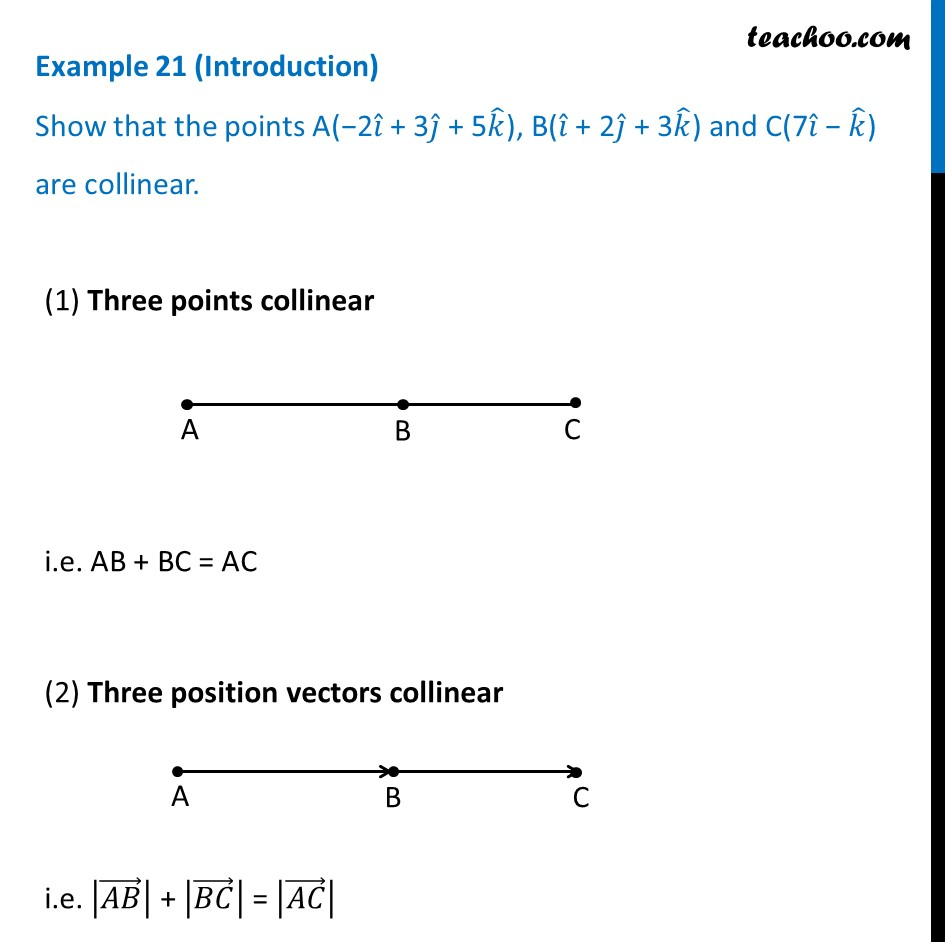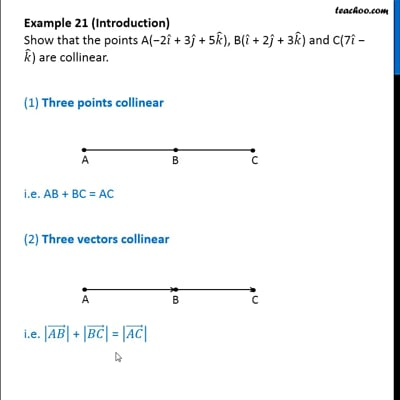This video is only available for Teachoo black users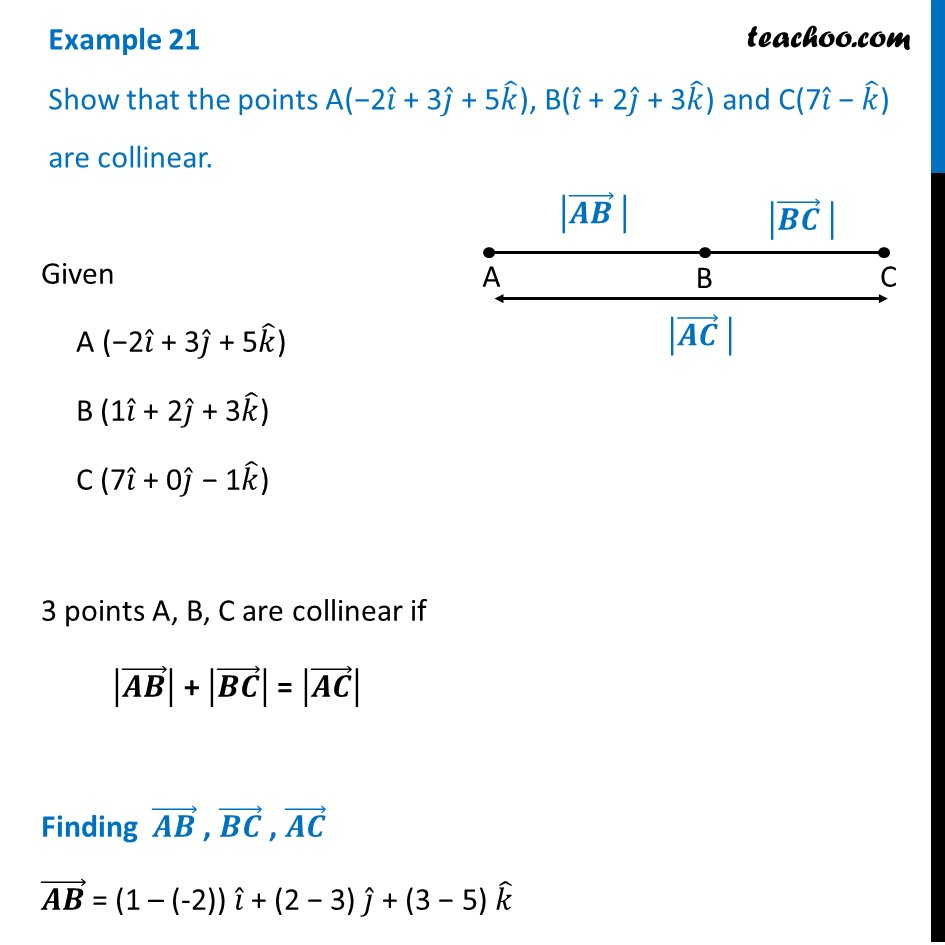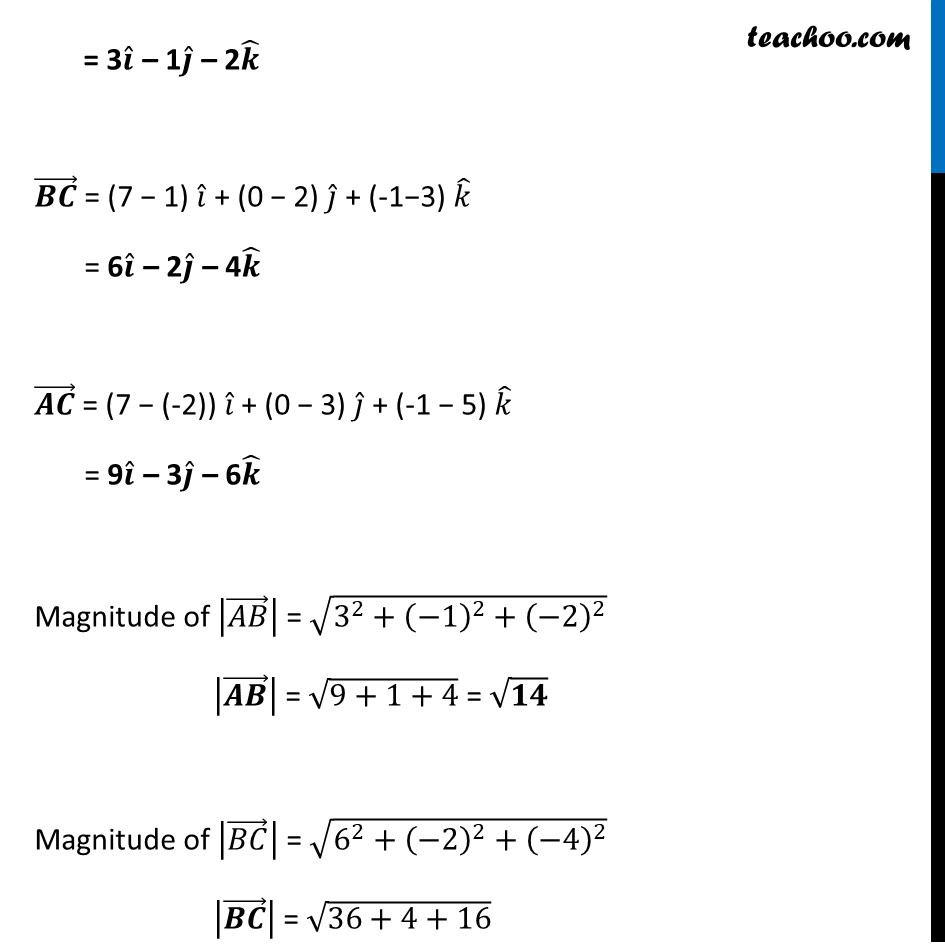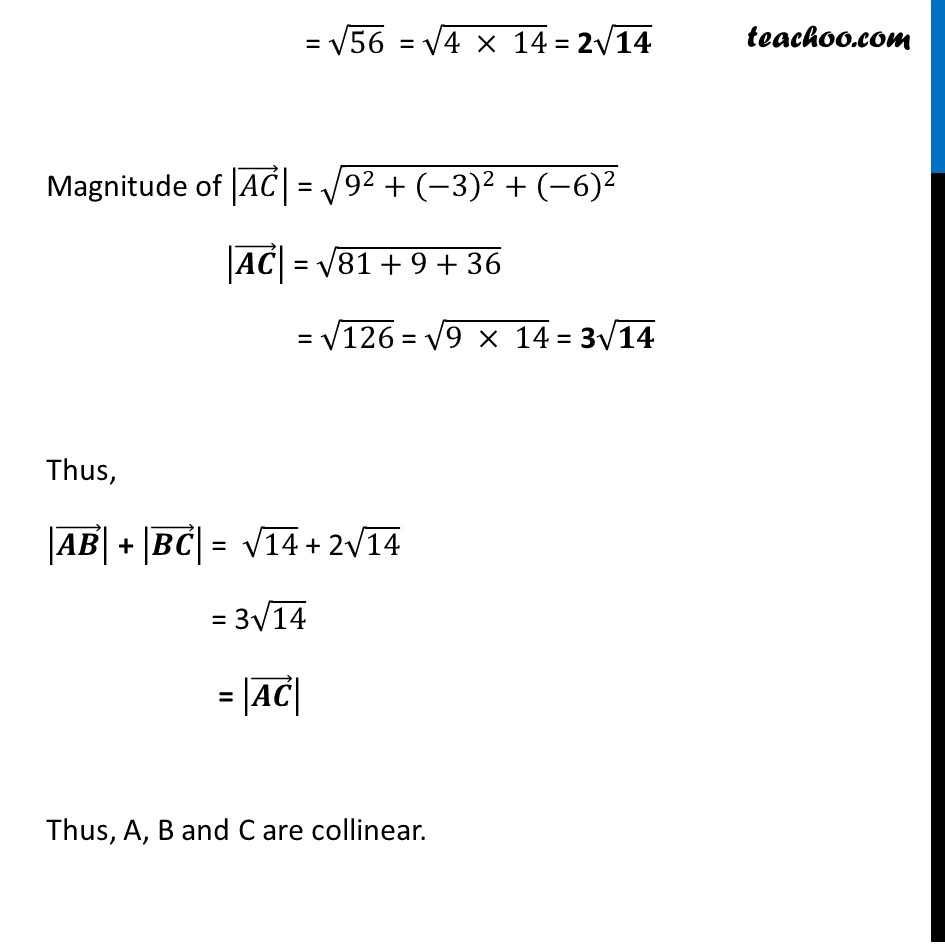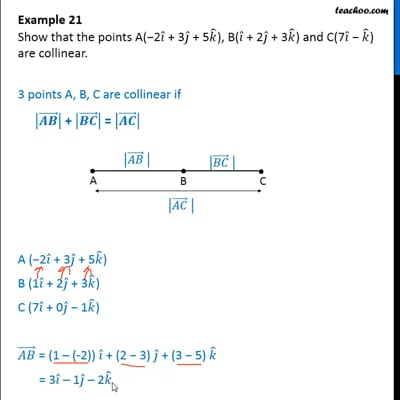This video is only available for Teachoo black users

Solve all your doubts with Teachoo Black (new monthly pack available now!)

### Transcript

Example 21 (Introduction) Show that the points A(−2𝑖 ̂ + 3𝑗 ̂ + 5𝑘 ̂), B(𝑖 ̂ + 2𝑗 ̂ + 3𝑘 ̂) and C(7𝑖 ̂ − 𝑘 ̂) are collinear. (1) Three points collinear i.e. AB + BC = AC (2) Three position vectors collinear i.e. |(𝐴𝐵) ⃗ | + |(𝐵𝐶) ⃗ | = |(𝐴𝐶) ⃗ | Example 21 Show that the points A(−2𝑖 ̂ + 3𝑗 ̂ + 5𝑘 ̂), B(𝑖 ̂ + 2𝑗 ̂ + 3𝑘 ̂) and C(7𝑖 ̂ − 𝑘 ̂) are collinear. Given A (−2𝑖 ̂ + 3𝑗 ̂ + 5𝑘 ̂) B (1𝑖 ̂ + 2𝑗 ̂ + 3𝑘 ̂) C (7𝑖 ̂ + 0𝑗 ̂ − 1𝑘 ̂) 3 points A, B, C are collinear if |(𝑨𝑩) ⃗ | + |(𝑩𝑪) ⃗ | = |(𝑨𝑪) ⃗ | Finding (𝑨𝑩) ⃗ , (𝑩𝑪) ⃗ , (𝑨𝑪) ⃗ (𝑨𝑩) ⃗ = (1 – (-2)) 𝑖 ̂ + (2 − 3) 𝑗 ̂ + (3 − 5) 𝑘 ̂ = 3𝒊 ̂ – 1𝒋 ̂ – 2𝒌 ̂ (𝑩𝑪) ⃗ = (7 − 1) 𝑖 ̂ + (0 − 2) 𝑗 ̂ + (-1−3) 𝑘 ̂ = 6𝒊 ̂ – 2𝒋 ̂ – 4𝒌 ̂ (𝑨𝑪) ⃗ = (7 − (-2)) 𝑖 ̂ + (0 − 3) 𝑗 ̂ + (-1 − 5) 𝑘 ̂ = 9𝒊 ̂ – 3𝒋 ̂ – 6𝒌 ̂ Magnitude of |(𝐴𝐵) ⃗ | = √(3^2+(−1)^2+(−2)^2 ) |(𝑨𝑩) ⃗ | = √(9+1+4) = √𝟏𝟒 Magnitude of |(𝐵𝐶) ⃗ | = √(6^2+(−2)^2+(−4)^2 ) |(𝑩𝑪) ⃗ | = √(36+4+16) = √56 = √(4 × 14) = 2√𝟏𝟒 Magnitude of |(𝐴𝐶) ⃗ | = √(9^2+(−3)^2+(−6)^2 ) |(𝑨𝑪) ⃗ | = √(81+9+36) = √126 = √(9 × 14) = 3√𝟏𝟒 Thus, |(𝑨𝑩) ⃗ | + |(𝑩𝑪) ⃗ | = √14 + 2√14 = 3√14 = |(𝑨𝑪) ⃗ | Thus, A, B and C are collinear.